# Learn the concepts of Linear Regression ( Theory as well as Code)

Regression is one of the most popular and probably the easiest machine learning algorithms. It comes under the supervised learning(label specified problems)

Regression basically helps out in finding the relation between independent variables to dependent variables. So what are dependent and independent variables?

## Dependent variables -:

Dependent variables represent a quantity whose value depends upon how the independent variables are changed or manipulated.

## Independent variables -:

Independent variables represent a quantity that is being manipulated.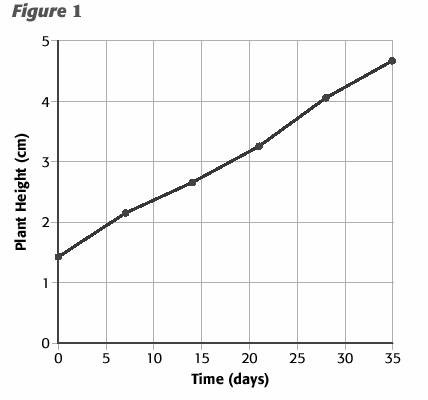In the above example, plant height depends upon the time(days) which represents that plant height(y-axis)is the dependent variable and on the other hand time(x-axis) is the independent variable.

The relation between dependent and independent variables is shown with the help of the linear line, often known as the best split line or decision boundary.

To understand linear regression more clearly we need a scatter plot of some data-set.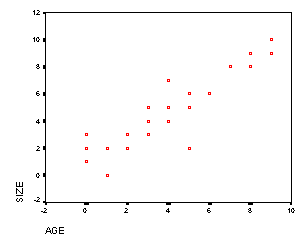The regression line on this data-set is represented as: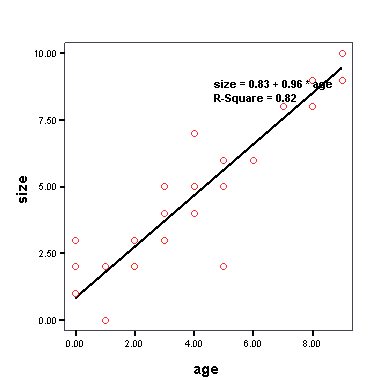Steps for making the best decision boundary

1. ## The equation for the regression line: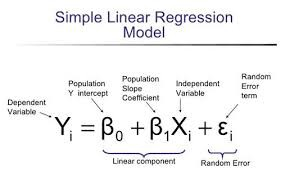This is just the normal linear line equation, generally denoted as ‘Y=mx + c’. This equation is also known as the hypothesis equation. Here Yi is predicted output and Xi is input or actual value.

## 2. Calculation of error:

Initially, the model will predict a line with a huge error, In order to train the model, we must calculate the error. Error is nothing but the difference between predicted and actual value. We need to make some error function to calculate the error.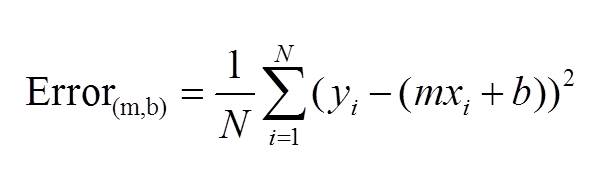Above is the error function of the regression model,Yi is the actual vertical distance, and (mxi + b) is the hypothesis equation.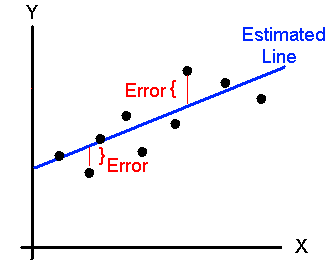error visualization

We have squared the error in order to get positive values and divided by N in order to ease the computation.

Once we have calculated the error, we desire to minimize the error so that we can conclude the best split line. We have a method known as gradient descent for minimization of error. Also, this gradient descent helps in the optimization of convex functions(which have only one local minima)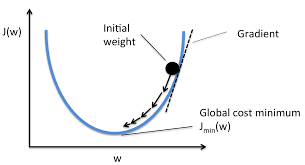fig 5

With the help of gradient descent, we compute the local minima of the distribution.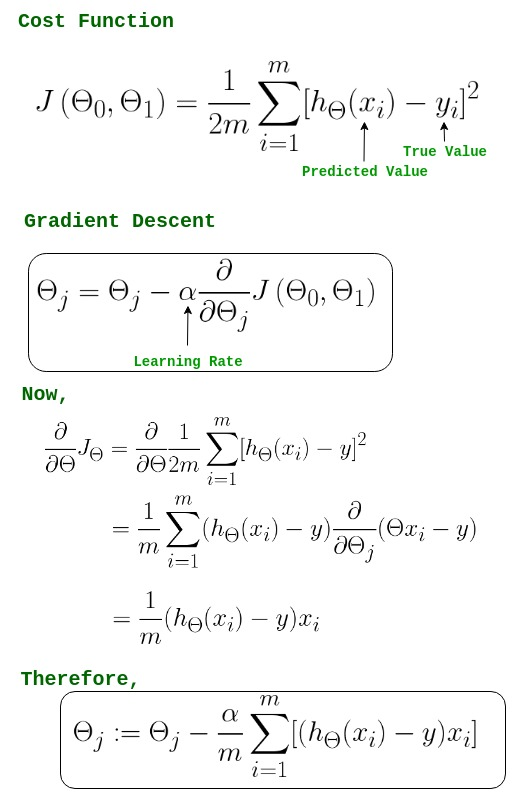fig 6

a is alpha or learning rate, the learning rate is nothing but steps taken from initial weight to local minima. More the learning rate, the larger will be the steps from initial weight to local minima and vice versa.

PSEUDO CODE:

1. Random value of initial weight
2. Measure how good the weight is -> error function
3. Minimization of error by gradient descent

Code :

import numpy as np
from matplotlib import pyplot as plt
from sklearn.datasets import make_regression

X, y = make_regression(n_samples=500, n_features=1, bias=4.5, noise=3.3)

print(X.shape, y.shape)

`[out] :(500, 1) (500,)    `

plt.figure()
plt.scatter(X[:,0], y)
plt.show()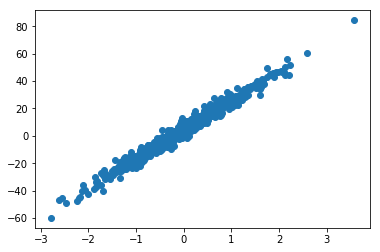class UniVariateLinearRegression:

def __init__(self, X, y):
self.X = X
self.y = y
self.coef = np.random.uniform(low=-1, high=1)
self.bias = np.random.random()

def compute_loss(self):
losses = []
for x,y in zip(self.X, self.y):
yhat = self.predict(x)
loss = (y - yhat)**2
losses.append(loss)

losses = np.array(losses)
return losses.sum() / (2 * self.X.shape)

for x,y in zip(self.X, self.y):
yhat = self.predict(x)

self.bias = self.bias - (learning_rate * gradients)
self.coef = self.coef - (learning_rate * gradients)
###

def predict(self, x):
return self.coef * x + self.bias

def score(self):
pass

def get_all_preds(self):
preds = []
for x in self.X:
preds.append(self.predict(x))
return preds

def train(self, losses, iterations=1, alpha=0.01):
for _ in range(iterations):
losses.append(self.compute_loss())
return losses

Inititalising Models

univariate = UniVariateLinearRegression(X, y)
losses = [univariate.compute_loss()]

losses

`[out] : [210.55936443361355]`

initial_preds = univariate.get_all_preds()

def plot_best_fit(X, y, preds, title=''):
plt.figure()
plt.title(title)
plt.scatter(X[:, 0], y)
plt.plot(X[:, 0], preds, 'r')
plt.show()

plot_best_fit(X, y, initial_preds, 'Initial Fit')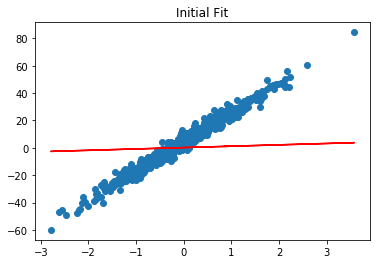Training Model

losses = univariate.train(losses, iterations=200, alpha=0.01)
losses[-10:]

```[out] : [6.10277969560787,
6.088057109386991,
6.0736136830183645,
6.059444120546921,
6.04554322654219,
6.0319059041891,
6.018527153415065,
6.005402069052658,
5.9925258390371745,
5.979893742638506]```

preds = univariate.get_all_preds()
plot_best_fit(X, y, preds)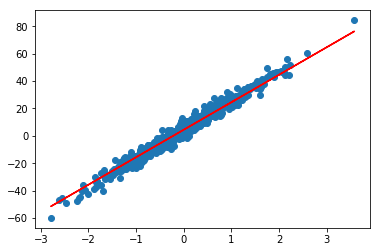plt.figure()
plt.plot(losses)
plt.show()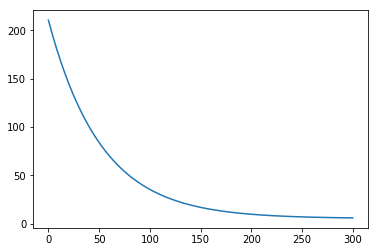### tanesh

Founder Of Aipoint, A very creative machine learning researcher that loves playing with the data.